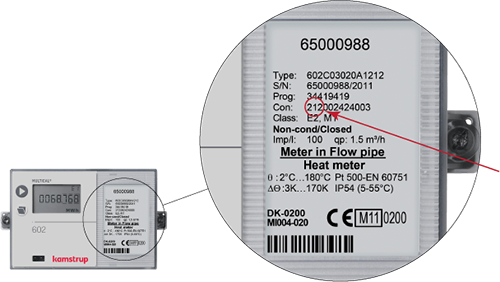#### Interactive User Guides

##### – Heat • Cooling • Water

MULTICAL® 66-CDE

MULTICAL® Compact

MULTICAL® 302

MULTICAL® 303

MULTICAL® 401

MULTICAL® 402

MULTICAL® 403

MULTICAL® 601

MULTICAL® 602

DDD = 110 / 210 / 410

DDD = 111 / 211 / 411

DDD = 112 / 212 / 412

DDD = 113 / 213 / 413

DDD = 114 / 214 / 414

DDD = 115 / 215 / 415

DDD = 116 / 216 / 416

DDD = 117 / 217 / 417

DDD = 117 / 217 / 417 GJ

DDD = 118 / 218 / 418

DDD = 119 / 219 / 419

DDD = 120 / 220 / 420

DDD = 121 / 221 / 421

DDD = 122 / 222 / 422

DDD = 123 / 223 / 423

DDD = 124 / 224 / 424

DDD = 125 / 225 / 425

DDD = 126 / 226 / 426

DDD = 127 / 227 / 427

DDD = 128 / 228 / 428

DDD = 129 / 229 / 429

DDD = 130 / 230 / 430

DDD = 131 / 231 / 431

DDD = 132 / 232 / 432

DDD = 133 / 233 / 433

DDD = 134 / 234 / 434

DDD = 137 / 237 / 437

DDD = 138 / 238 / 438

DDD = 142 / 242 / 442

DDD = 143 / 243 / 443

DDD = 156 / 256 / 456

DDD = 157 / 257 / 457

DDD = 158 / 258 / 458

DDD = 159 / 259 / 459

DDD = 182 / 282 / 482

DDD = 183 / 283 / 483

DDD = 248

DDD = 310

DDD = 311

DDD = 312

DDD = 314

DDD = 315

DDD = 327

DDD = 510

DDD = 513

DDD = 514

DDD = 515

DDD = 516

DDD = 610

DDD = 611

DDD = 612

DDD = 614

DDD = 615

DDD = 616

DDD = 623

DDD = 710 / 810

DDD = 711 / 811

DDD = 712 / 812

DDD = 713 / 813

DDD = 714 / 814

DDD = 723 / 823

DDD = 756 / 856

DDD = 816

DDD = 910

DDD = 912

DDD = 913

DDD = 914

DDD = 915

DDD = 916

DDD = 917

DDD = 918

DDD = 919

DDD = 920

DDD = 921

DDD = 922

DDD = 923

DDD = 977

DDD = 978

DDD = 979

DDD = 995

DDD = 996

DDD = 997

DDD = 998

MULTICAL® 603

MULTICAL® 801

MULTICAL® 803

SVM S6

MULTICAL® 61

MULTICAL® 62

# MULTICAL® 602

## Here you can see how your meter works. Find out with which DDD code your meter has been programmed, and find it on the list in the menu to the left.